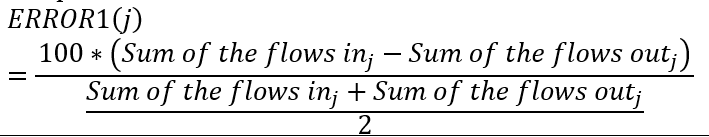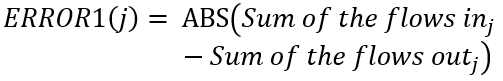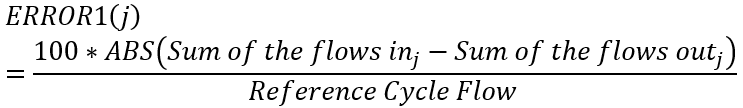# Solver Convergence

This Panel Consists of Various Tolerance & Convergence criteria for Flow Simulator solver runs.

 Parameter Description Flow Simulator Chamber Initialization (INITMODE) Specifies method of the initial internal (non-boundary) chamber and element value seeding for the Flow Simulator solver. Static pressures and total temperatures are seeded to the Flow Simulator solver for all of the methods below. 1: Initial values in the chamber data section of the model database file will be used as the initial guess for starting the Flow Simulator solution. 2: Compressible flow initializer will be called to produce improved initial values. Element flow rates are also seeded to the Flow Simulator solver. 10: If post-data (from a previously Flow Simulator solution) is available at the bottom of the database file, then the post-data will be used for seeding; otherwise the INITMODE = 1 option will be carried out. 20: If post-data is available, then post-data will be used; otherwise the INITMODE = 2 option will be carried out. Default value is 0, which is interpreted as 1 by Flow Simulator. The Auto-Initialize function sets the INITMODE to 2 Solver Selection Solver Selection 0: Standard Solver (DEFAULT) 1: Robust Multi-Grid Solver 2: Transitional Solver (Default value = 0.0) Maximum Number of Iterations Maximum allowable number of flow iterations to reach a converged steady-state solution. Flow Simulator will iterate up to this number of times in search of the converged solution of the flow network. (Default value = 100) Local Mass Continuity Tolerance #1 (% of Chamber Through-Flow) Tol1 Maximum acceptable flow error in any non-boundary chamber for the model to be considered 'converged' where flow error in chamber 'j' is defined by the equation:This is mass balance as a percentage of the average through flow. By default , TOL1 is the primary flow error tolerance variable. (Default TOL1 = 1%) But should be reduced as far as possible (0.1% or 0.01% are good). Global Mass Continuity Tolerance #2 (% of Network Through-Flow) Tol2 Maximum acceptable flow error in any non-boundary chamber for the model to be considered 'converged' with flow error in chamber 'j' based on the following equation:This is mass balance as a percentage of the total flow into and out of all boundary chambers. By default, TOL2 is the secondary flow error tolerance variable. Since ERROR2 is based on the boundary flows rather than the local chamber, TOL2 should be more easily satisfied than the same value of TOL1. In those cases where some chambers have small flows, which are possibly unimportant to the user, TOL2 is a useful means of solving a model to within acceptable engineering accuracy. (Default value = 1000.) TOL2 may also be used as an alternative to TOL1 when the variable TOL2_CHAM is set. The use of TOL2 in this option is explained in the TOL2_CHAM description. Global Mass Imbalance Based on Absolute Mass Flow Rate (Lbm/sec) Maximum acceptable flow error in any non-boundary chamber for the model to be considered 'converged' where flow error in chamber 'j' is defined by the equationError1 can be the most severe since the Error can be large if the chamber thru-flow is very low even if the imbalance is low in terms of lbm/sec. TOL3 can be used if you model has convergence problems using Error1. Global Mass Imbalance % of Ref Flow Tol4 Maximum acceptable flow error in any non-boundary chamber for the model to be considered 'converged' where flow error in chamber 'j' is defined by the equationThe Reference flow rate for Error4 is set in the Analysis Setup → Reference Conditions → Reference Flow Rate (lbm/sec) User can use the option they feel gives them accurate results and good run time. Transition Iteration Iteration when solver transitions from Standard Solver to Robust Solver if using the Transitional Solver option (SOLVER_TYPE=2). (Default value = 0.0) Vortex Pressure Ratio Tolerance The maximum absolute difference between up-to-date vortex pressure ratio and damped vortex pressure ratio must be less than VTX_PR_TOL for the model to be declared converged. Used for Robust Multi Grid & Transitional Solver (Default value = 1E-5) Dynamic Pressure Ratio Tolerance The maximum absolute difference between up-to-date dynamic pressure ratio (PTS/PS) and damped dynamic pressure ratio must be less than DYN_PR_TOL for the model to be declared converged. Used for Robust Multi Grid & Transitional Solver (Default value = 1E-5) Chamber that uses Special Tolerance #2 Flag that activates the alternative use of the convergence input variable TOL2. The purpose of this variable is to allow declaration of convergence of models that, based on TOL1, would converge except for local chambers that are extremely slow (or impossible) to converge but are in areas of the model not critical to the analysis or in low flow areas where large percentage mass discontinuities are not critical. The approach for its use is to select a chamber that is normally slow to converge, as judged by ERROR1, but is not in the “trouble” zone. The idea being that when the selected chamber meets convergence tolerance, all the important chambers are converged. The selection of an appropriate chamber can be tricky so this option should be used with care. TOL2 is then input as a value substantially smaller than TOL1 and TOL2_CHAM is set to plus or minus AAAA.BBB where AAAA is the number of the selected reference chamber and BBB is the iteration after which the model will be allowed to declare convergence if ERROR1 in the reference chamber is less than TOL2. This declaration of convergence will be independent of convergence in all other chambers. Convergence would also be declared if all chambers meet TOL1 tolerance with their ERROR1 values. With positive values of TOL2_CHAM, convergence can only be declared if all cavities (if any) have converged. Negative values serve as a flag that declaration of convergence is independent of cavities. (Default value = 0000.000 means that the TOL2_CHAM criteria is not used) Total Pressure Tolerance (% Change between Iterations) Convergence tolerance for the maximum percentage change in total pressure at any chamber from one iteration step to the next. PTTOL applies only to momentum and inertial chambers. The model will not converge until this tolerance is satisfied. (Default value = 0.01%) Theta Angle Tolerance (Degrees Change between Iterations) Convergence tolerance for the maximum allowable change in the fluid THETA flow Angle at any chamber from one iteration step to the next. THTOL applies only to momentum and inertial chambers. The model will not converge until this tolerance is satisfied. (Default value = 0.1 deg) Tolerance for Radius Consistency Radial tolerance (inches) for checking consistency of radius data for: Elements connected to the same chamber Vortex radii with associated cavity inflow and outflow radii (in). Warning messages are issued for elements that do not meet the tolerance, but the solution process is not stopped. (Default value = 0.01; Minimum = 0.001) Temperature Tolerance (degF) Convergence tolerance for the maximum change in temperature (deg F) at any chamber. The model will not converge until this tolerance is satisfied. (Default value = 1.0 deg F) Lower Partial Pressure Limit (where species is ignored) Lower partial pressure limit whereby the fluid composition of a particular species is no longer considered in properties calculations. For example, if gas properties for a mixture of air and steam is needed for a calculation and the partial pressure of steam is less than this limit, the properties will be based on pure air. (Default value = 0.1 psia) Diffuser Cp Tolerance Tolerance for iteration-to-iteration change in Cp (Static Pressure Recovery Coefficient) for Diffuser elements. (Default value = 0.001) Angular Momentum Imbalance Cavity solution tolerance for net wall torque / angular momentum change. (Default value = 1.0E-04) Torque Integration Method Cavity torque integration method. (Default value = 1) Swirl Change Tolerance Tolerance for swirl change between cavity runs. If swirl change is greater than XK_CHANGE_TOL, then Flow Simulator will continue running. (Default value = 0.02) Max Iterations Maximum number of iterations for cavity solver convergence. (Default value = 200) Cavity Surface Tolerance Tolerance value to define a surface segment as either horizontal or vertical (in). (Default value = 0.0012) Max Cavity Interval Maximum number of Flow Simulator solver iterations between cavity solver runs. If the limit is reach, the cavity solver is forced to run. (Default value = 50) Max Cavity Runs Maximum number of cavity solver runs. (Default value = 99999) Cavity First Run Iteration Limit Number of iterations at the start when CAV_FIRST_RUN_ERR_LIMIT applies. (Default value = 50) Cavity First Run Error Limit Maximum flow balance error to trigger a cavity run. Used when number of Flow Simulator iterations is less than or equal to CAV_FIRST_RUN_ITER_LIMIT. (Default value = 100%) Cavity Run Sec. Limit Factor Maximum flow balance error to trigger a cavity run. Used when the number of Flow Simulator iterations is greater than CAV_FIRST_RUN_ITER_LIMIT. (Default value = 2.5%) Max Cavity Run after Flow Convergence Maximum number of times cavity will run after flow balance is achieved before giving up. (Default value = 10) Cavity Flow Continuity Run Limit (%) Value of maximum error (cavity chamber flow balance) allowed above which the individual cavity is not run. (Default value = 50.0%) Maximum number of thermal iterations Maximum allowable number of iterations to reach a converged solution for the thermal network solver. Flow Simulator will iterate up to this number of times in search of the converged solution of the thermal network. (Default value = 100.) Thermal network heat imbalance tolerance (Btu/s) Convergence tolerance for the maximum heat imbalance in Btu/s in a thermal network. (Default value = 0.00001 Btu/s) Thermal Network Solution Matrix Thermal Network Solver Selection 0: Linear System Solver (Default) 1: Sparse Matrix Solver